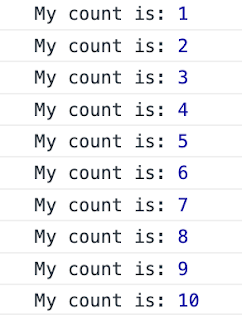# Javascript: How to print numbers from 1 to 10 using javascript setInterval?

Javascript: How to print numbers from 1 to 10 using javascript setInterval?

Solution: This is basic interview questions.

setInterval() method is used to call a function or evaluate an expression at specified intervals (in milliseconds). For example, let's log a message after 1 seconds using setInterval method,

```setInterval(function () {
console.log("Hello Javascript");
}, 1000);```
`Solution for above Question is:`
```let i =1;
let basicint = setInterval(function () {
console.log("My count is:",i);
i++;
if(i>10){
clearInterval(basicint); // Here clearing the interval after 10 iterations
}
}, 1000);```
```

```
`Finally the out is:`
``

More